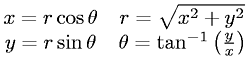Equations > Geometry > Coordinate Systems > Cartesian to Polar Coordinates (2-D)

Cartesian to Polar Coordinates (2-D)Latex Code:

MathML Code:

 $\begin{array}{cc}\hfill x=r\mathrm{cos}\theta \hfill & \hfill r=\sqrt{{x}^{2}+{y}^{2}}\hfill \\ \hfill y=r\mathrm{sin}\theta \hfill & \hfill \theta ={\mathrm{tan}}^{-1}\left(\fracyx\right)\hfill \end{array}$

MathType 5.0: